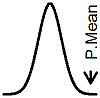P.Mean: Why the least squares regression line has to pass through XBAR, YBAR (created 2010-10-01).An issue came up about whether the least squares regression line has to pass through the point (XBAR,YBAR), where the terms XBAR and YBAR represent the arithmetic mean of the independent and dependent variables, respectively. The line does have to pass through those two points and it is easy to show why.

The least squares estimates represent the minimum value for the following sum:In basic calculus, we know that the minimum occurs at a point where both partial derivatives are equal to zero.These are the famous normal equations. You can simplify the first normal equation toand divide both sides of the equation by n to getNow there is an alternate way of visualizing the least squares regression line. If you center the X and Y values by subtracting their respective means, the new regression line has to go through the point (0,0), implying that the intercept for the centered data has to be zero. This means that the least squares criteria can be written aswhich can be rewritten asThe value of b that minimizes this equations is a weighted average of n slope values where the slopesrepresent the estimated slope when you join each data point to the mean of all the data points. The weightsinsure that the points further from the center of the data get greater emphasis. Here's a picture of what is going on.Notice that the points close to the middle have very bad slopes (meaning quite discrepant from the remaining slopes). But this is okay because those points get very little weight in the weighted average.

Pretty cool, huh?This work is licensed under a Creative Commons Attribution 3.0 United States License. This page was written by Steve Simon and was last modified on 2017-06-15. Need more information? I have a page with general help resources. You can also browse for pages similar to this one at Category: Linear regression.